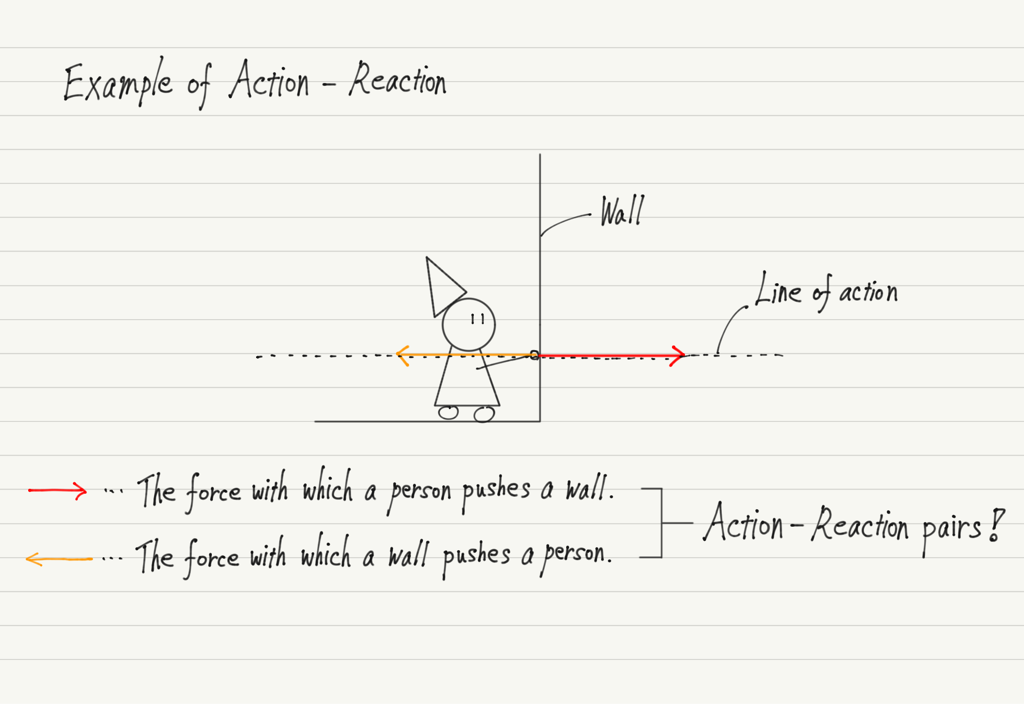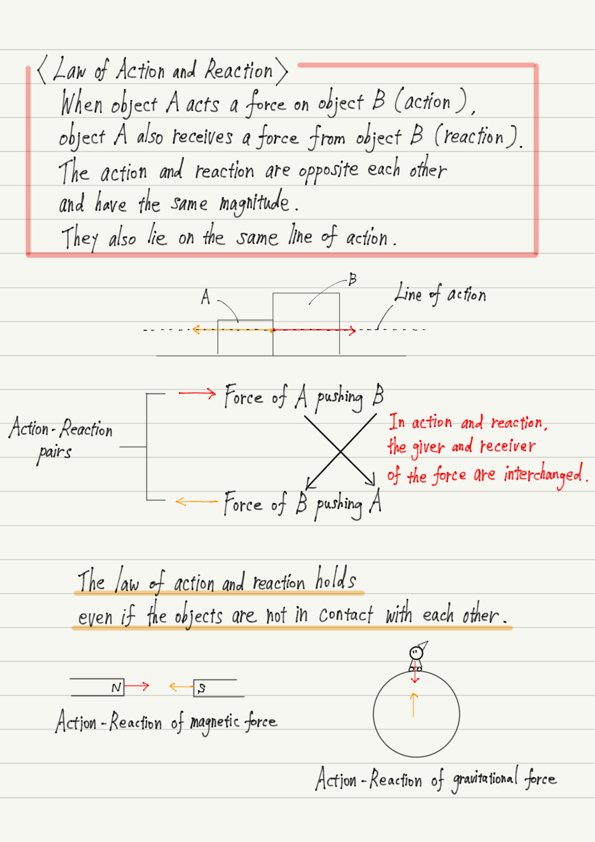# Law of Action and Reaction

In the last lecture, we learned about the first law of motion.
This time, I would like to skip the second law and explain the third law.

## Newton’s third law of motion

The Newton’s third law of motion is also known as the “Law of action and reaction”.
The assertion of this law is as follows.

“When object A acts a force on object B (action), object A also receives a force from object B (reaction). The action and reaction are opposite each other and have the same magnitude. They also lie on the same line of action.”

This is all about the law of action and reaction. As you can see, this is a law about forces.
Despite the name “third law of motion,” there is no reference to motion.

## Deciphering the law of action and reaction

Now, let me explain using the example of a casual action of “pushing a wall”.
Try actually pushing a nearby wall.

When you push a wall, you tend to think, “I am acting a force and unilaterally pushing the wall.”
But in fact, the law of action and reaction asserts that “as you push the wall, you are also being pushed by the wall”!Note that action and reaction are not cause and effect, but “pairs of forces that appear simultaneously”.

Since the magnitude of the reaction is equal to the magnitude of the action, if you push on a wall with a force of 50 N, you will be pushed back by the wall with a force of 50 N, and if you push with a force of 100 N, you will be pushed back by the wall with a force of 100 N.

## You are pulling the earth now!

One last note. The law of action and reaction holds even if the objects are not in contact with each other!

For example, in a magnet, the N and S poles are attracted to each other, but at the same time the N pole is pulled toward the S pole, the S pole is also pulled toward the N pole. This is exactly action and reaction.

Like magnetic force, gravitational force has a reaction.
As you are reading this sentence, you are being pulled by the earth’s gravity, but what will be the reaction?

Since gravitational force is “the force with which the earth pulls you,” this reaction is “the force with which you pull the earth!”
And its magnitude is equal to the gravitational force you receive from the earth.

Suppose you weigh 50 kg, you are pulling the earth at all times with a force of 490 N (mg = 50 ✕ 9.8)!

One of the interesting things about the laws of physics is that they hold true at different scales.

Isn’t it inspiring to know that the law of action and reaction exists between you and the earth?

## Summary of this lecture## Next Time

In the next lecture, we will study the remaining one of the Newton’s three laws, the second law of motion!

https://www.yukimura-physics.com/entry/dyn-f21

error: Content is protected !!